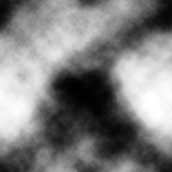# Fast Marble Texture Algorithm

Hamburg (Germany), the 29th April 1997. Written by Nils Pipenbrinck aka Submissive/Cubic & \$eeN## Introduction

I've been asked several times how I did the nice marble-style textures in our winning 64k intro Lasse Rein Bøng. Well like almost all Effects it's not too hard, but you need the idea. I'll explain how the algorithm works and will also provide some example code.

## Theory of Displacements

The algorithm is pretty easy. Technically it takes an input image and transforms it into an output image by altering the position of every pixel a little bit. The key-element of this algorithm is building a table and a displacement-map to calculate the new position of the pixels. I chose an ordinary fractal-plasma picture both as the source image and as the displacement map. However, the algorithm works well with other pictures as long as you don't have rapid changes of pixel-values.

Ok, now let's put it together: We have a displacement map. We need two tables which say how the pixels will be displaced. I used a very simple formula to generate these tables:

```for (int i=0; i<256; i++)
{
sval[i]=-scale*sin((float)i/(float)turbulence);
cval[i]=scale*cos((float)i/(float)turbulence);
}```

Scale controls how far the pixels may be displaced while turbulence controls the local turbulence. You have to experiment with the values to get a feeling how they work together.
And we need some code to actually displace the pixels:

```for (int x=0; x<256; x++)
for (int y=0; y<200; y++)
{
unsigned char xx=(x+sval[displacementmap[x+(y<<8)]]);
unsigned char yy=(y+cval[displacementmap[x+(y<<8)]]);
dest[x+(y<<8)]=source[xx+(yy<<8)];
}```

That's all, it's tiny, it's pretty, it works.

## Plasmas? What's that?A plasma is a generated bitmap which has some properties:

• It has a wide range of different color-values.
• The difference between two neighbouring pixels is small
• The bitmap is seamless (tileable). That means the left side fits with the right, and the upper with the bottom.

Plasmas are generated using a recursive algorithm. I won't explain how to do this because there are enough tutorials on this available. If you have no idea how to do it you might download an .asm file here. This code compiles with TASM and is made for the flat memory model. Please note that the algorithm is not optimized for speed but for size.

## C(++) Example Code

This program puts it all together. It's written for Watcom C++ (flat memory model) and needs some changes for other compilers. Final thing to note: I used two different plasmas as displacement maps. One for the X, and one for the Y displacement. This gives better results in some cases.

```#include "subplasm.h"
#include <conio.h>
#include <math.h>
#include <mem.h>
#include <malloc.h>
#include <stdlib.h>

// Code to set the Video-Mode
void SetBiosMode (short int);
#pragma aux SetBiosMode=   \
"int 0x10"         \
parm [ax]          \
modify [ax];

// This is the Marble-Generator
void marble (unsigned char *source, unsigned char *dest, float scale, float turbulence, unsigned char *xrpage, unsigned char *yrpage)
{
int cval;
int sval;
for (int i=0; i<256; i++)
{
sval[i]=-scale*sin((float)i/(float)turbulence);
cval[i]=scale*cos((float)i/(float)turbulence);
}
for (int x=0; x<256; x++)
for (int y=0; y<200; y++)
{
unsigned char xx=(x+sval[xrpage[x+(y<<8)]]);
unsigned char yy=(y+cval[yrpage[x+(y<<8)]]);
dest[x+(y<<8)]=source[xx+(yy<<8)];
}
}

// Some static data. Not really interesting
char test;
char source;
char *video = (char *) 0xa0000;

// Code to blit a marble to the screen
void inline display (void)
{
for (int y=0; y<200; y++)
{
memmove(&video[320*y], &test[y], 255);
memmove(&video[320*y+255], &test[y], (320-256));
}
}

// and the main program
void main (void)
{
char *xrpage = (char *) malloc (0x10000);
char *yrpage = (char *) malloc (0x10000);

// Generate Source Images (we use a different plasma for x and y
// displacement. That makes the marbles more weired!
makeplasma((void *) xrpage, 24401, 420);
makeplasma((void *) yrpage, 55400, 420);
makeplasma ((void *) source, 63703, 750);

// Set Video Mode and a grayscale Palette
SetBiosMode (0x13);
outp (0x3c8, 0);
for (int c=0; c<256; c++)
{
outp (0x3c9, c>>2);
outp (0x3c9, c>>2);
outp (0x3c9, c>>2);
}

// Animate the Marble Textures
for (int scale=0;; scale+=2)
{
memcpy (test, source, 0x10000);
marble   ((unsigned char *) source,
(unsigned char *) test,
scale,
120.0,
(unsigned char *) xrpage,
(unsigned char *) yrpage);
display();
if (kbhit()) break;
}
SetBiosMode (0x03);
free (xrpage);
free (yrpage);
}```

## Final Words

I rewrote most of the code while formatting it in html, so I had no chance to compile it. There might be one or two bugs in it but I guess you're clever enough to fix them. This algorithm is very useful for intros and programs where the size of the code is an issue. I haven't done much with this algorithm. I think there are many more ways to use it and this is the primary reason why I release it. I hope that some of you will take the idea and make some stunning great textures. If you use the code learn from it! Don't simply copy it and put it into your program. It would also be nice if you put me on your credit list (I'n not really hungry 'bout being greeted, but it would be nice to see that you respect my work and my ideas).